# FIR Decimator

Finite impulse response (FIR) decimation filter

• Library:
• DSP HDL Toolbox / Filtering

•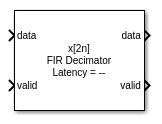## Description

The FIR Decimator block implements a single-rate polyphase FIR decimation filter that is optimized for HDL code generation. The block provides a hardware-friendly interface with input and output control signals. To provide a cycle-accurate simulation of the generated HDL code, the block models architectural latency including pipeline registers and resource sharing.

The block accepts scalar or vector input. When you use vector input and the vector size is less than the decimation factor, the decimation factor must be an integer multiple of the vector size. In this case, the output is scalar and an output valid signal indicates which samples are valid after decimation. The output data is valid every DecimationFactor/VectorSize samples. The waveform shows an input vector of four samples and a decimation factor of eight. The output data is a scalar that is valid every second cycle.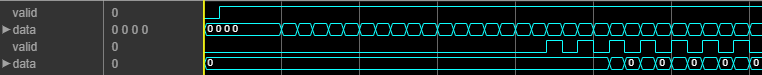When you use vector input and the vector size is greater than the decimation factor, the vector size must be an integer multiple of the decimation factor. In this case, the output is a vector of VectorSize/DecimationFactor samples. The waveform shows an input vector of eight samples and a decimation factor of four. The output data is a vector of two samples on every cycle.The block provides two filter structures. The direct form systolic architecture provides an implementation that makes efficient use of Intel® and Xilinx® DSP blocks. This architecture can be fully parallel or serial. To use a serial architecture, the input samples must be spaced out with a regular number of invalid cycles between the valid samples. The direct form transposed architecture is a fully parallel implementation that is suitable for FPGA and ASIC applications. For a filter implementation that matches multipliers, pipeline registers, and pre-adders to the DSP configuration of your FPGA vendor, specify your target device when you generate HDL code.

All filter structures optimize hardware resources by sharing multipliers for symmetric or antisymmetric filters and by removing the multipliers for zero-valued coefficients such as in half-band filters and Hilbert transforms.

The block implements one filter for each sample in the input vector. The block then shares this filter between the polyphase subfilters by interleaving the subfilter coefficients in time.

## Ports

### Input

expand all

Input data must be a real- or complex-valued scalar or vector. When you use vector input and the vector size is less than the decimation factor, the decimation factor must be an integer multiple of the vector size. When you use vector input and the vector size is greater than the decimation factor, the vector size must be an integer multiple of the decimation factor. The vector size must be less than or equal to 64.

When the input data type is an integer type or a fixed-point type, the block uses fixed-point arithmetic for internal calculations.

`double` and `single` data types are supported for simulation, but not for HDL code generation.

Data Types: `fixed point` | `single` | `double` | `int8` | `int16` | `int32` | `uint8` | `uint16` | `uint32`
Complex Number Support: Yes

Control signal that indicates if the input data is valid. When valid is `1` (`true`), the block captures the values from the input data port. When valid is `0` (`false`), the block ignores the values from the input data port.

Data Types: `Boolean`

Control signal that clears internal states. When reset is `1` (`true`), the block stops the current calculation and clears internal states. When the reset is `0` (`false`) and the input valid is `1` (`true`), the block captures data for processing.

For more reset considerations, see the Reset Signal section on the Hardware Control Signals page.

#### Dependencies

To enable this port, on the Control Ports tab, select Enable reset input port.

Data Types: `Boolean`

### Output

expand all

Filtered output data, returned as a real- or complex-valued scalar. When the input data type is a floating-point type, the output data inherits the data type of the input data. When the input data type is an integer type or a fixed-point type, the Output parameter on the Data Types tab specifies the output data type.

The output valid signal indicates which samples are valid after decimation. When the input vector size is greater than the decimation factor, the output is a vector of VectorSize/DecimationFactor samples.

Data Types: `fixed point` | `single` | `double`
Complex Number Support: Yes

Control signal that indicates if the data from the output data port is valid. When valid is `1` (`true`), the block returns valid data from the output data port. When valid is `0` (`false`), the values from the output data port are not valid.

Data Types: `Boolean`

## Parameters

expand all

### Main

FIR filter coefficients, specified as a real- or complex-valued vector. You can specify the vector as a workspace variable or as a call to a filter design function. When the input data type is a floating-point type, the block casts the coefficients to the same data type as the input. When the input data type is an integer type or a fixed-point type, you can set the data type for the coefficients on the Data Types tab.

Example: ```firpm(30,[0 0.1 0.2 0.5]*2,[1 1 0 0])``` defines coefficients using a linear-phase filter design function.

Data Types: `single` | `double` | `int8` | `int16` | `int32` | `uint8` | `uint16` | `uint32`
Complex Number Support: Yes

The block implements a polyphase decomposition filter by using Discrete FIR Filter blocks. Both structures share resources by interleaving the subfilter coefficients over one filter implementation for each sample in the input vector. Specify the HDL filter architecture as one of these structures:

• ```Direct form systolic``` — This architecture provides a parallel or partly serial filter implementation that makes efficient use of Intel and Xilinx DSP blocks. For a partly serial implementation, specify a value greater than 1 for the Minimum number of cycles between valid input samples parameter. You cannot use frame-based input with the partly serial architecture.

When Minimum number of cycles between valid input samples is greater than 1, the block chooses a filter architecture that results in the fewest multipliers. If N allows for a single multiplier in each subfilter, then the block implements a single serial filter and decimates the output samples.

• ```Direct form transposed``` — This architecture is a fully parallel implementation that is suitable for FPGA and ASIC applications.

All implementations share resources by interleaving the subfilter coefficients over one filter implementation for each sample in the input vector.

The block implements a polyphase decomposition filter using Discrete FIR Filter blocks. For architecture details, see FIR Filter Architectures for FPGAs and ASICs.

Specify an integer decimation factor greater than two. When you use vector input and the vector size is less than the decimation factor, the decimation factor must be an integer multiple of the vector size. When you use vector input and the vector size is greater than the decimation factor, the vector size must be an integer multiple of the decimation factor.

Serialization requirement for input timing, specified as a positive integer. This parameter represents N, the minimum number of cycles between valid input samples. To implement a fully serial architecture, set Minimum number of cycles between valid input samples greater than the filter length, L, or to `Inf`.

The block applies coefficient optimizations before serialization, so the sharing factor of the final filter can be lower than the number of cycles that you specified.

#### Dependencies

To enable this parameter, set Filter structure to ```Direct form systolic```.

You cannot use frame-based input with Minimum number of cycles between valid input samples greater than 1.

### Data Types

Rounding mode for type-casting the output to the data type specified by the Output parameter. When the input data type is floating point, the block ignores this parameter. For more details, see Rounding Modes.

Overflow handling for type-casting the output to the data type specified by the Output parameter. When the input data type is floating point, the block ignores this parameter. For more details, see Overflow Handling.

The block casts the filter coefficients to this data type. The quantization rounds to the nearest representable value and saturates on overflow. When the input data type is floating point, the block ignores this parameter.

The recommended data type for this parameter is ```Inherit: Same word length as input```.

The block returns a warning or error if either of these conditions occur.

• The coefficients data type does not have enough fractional length to represent the coefficients accurately.

• The coefficients data type is unsigned, and the coefficients include negative values.

The block casts the output of the filter to this data type. The quantization uses the settings of the Rounding mode and Overflow mode parameters. When the input data type is floating point, the block ignores this parameter.

The block increases the word length for full precision inside each filter tap and casts the final output to the specified type. The maximum final internal data type (WF) depends on the input data type (WI), the coefficient data type (WC), and the number of coefficients (L) and is given by

```WF = WI + WC + ceil(log2(L))```.

Because the coefficient values limit the potential growth, usually the actual full-precision internal word length is smaller than WF.

### Control Ports

Select this parameter to enable the reset input port. The reset signal implements a local synchronous reset of the data path registers.

For more reset considerations, see the Reset Signal section on the Hardware Control Signals page.

Select this parameter to connect the generated HDL global reset signal to the data path registers. This parameter does not change the appearance of the block or modify simulation behavior in Simulink®. When you clear this parameter, the generated HDL global reset clears only the control path registers. The generated HDL global reset can be synchronous or asynchronous depending on the HDL Code Generation > Global Settings > Reset type parameter in the model Configuration Parameters.

For more reset considerations, see the Reset Signal section on the Hardware Control Signals page.

## Algorithms

expand all

The block implements a polyphase filter bank where the filter coefficients are decomposed into DecimationFactor subfilters. If the filter length is not divisible by the Decimation factor parameter value, then the block zero-pads the coefficients. When your input is regularly spaced, with two or more cycles between valid samples, as indicated by the Minimum number of cycles between valid input samples parameter, the filter can share multiplier resources in time.

This flow chart shows which filter architectures result from your parameter settings. It also shows the number of multipliers used by the filter implementation. The filter architecture depends on the input frame size, V, the decimation factor, R, the number of cycles between valid input samples, N, and the number of filter coefficients, L. The architectures are in order from lowest resource use on the left, to higher resources on the right. The higher resource architectures are trading off resource use for higher throughput. Each architecture is described below the flow chart.

The number of multipliers shown in the flow chart is for filters with real input and real coefficients. For complex input, the filter uses three times as many multipliers.• Architecture 1 — Fully parallel one-tap interleaved polyphase filter bank.

When DecimationFactor is greater than the filter length, for any value of NumCycles, the filter becomes a single-tap filter with interleaved coefficients, and uses a single multiplier.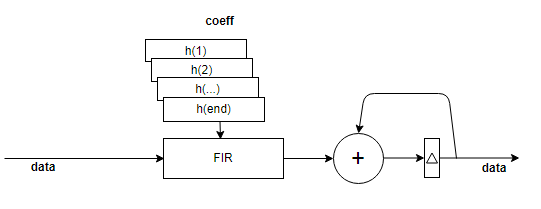• Architecture 2 — Single fully serial filter.

When the filter has NumCycles greater than the number of filter coefficients, the block implements a single fully serial filter and decimates the output samples by the decimation factor. This serial filter uses one multiplier.• Architecture 3 — Partly serial polyphase filter bank.

When the filter has NumCycles greater than one and less than the number of filter coefficients, the block implements a polyphase filter with DecimationFactor subfilters. This diagram shows input data with a valid sample every second cycle and a DecimationFactor of `4`. The output data has one valid sample every eight cycles. This filter implementation uses FilterLength/NumCycles multipliers.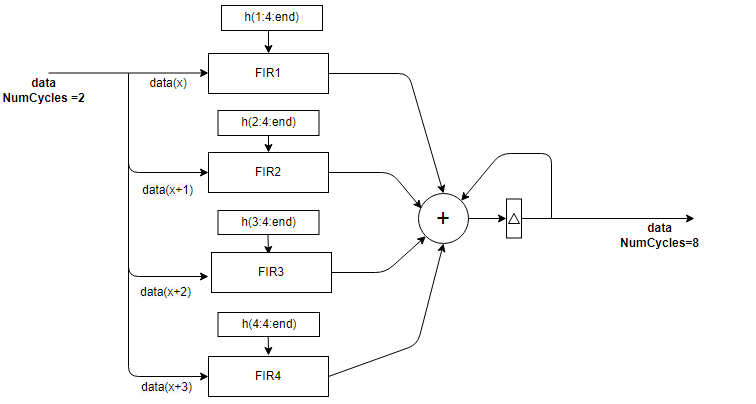• Architecture 4 — Fully parallel polyphase interleaved filter bank (scalar).

The diagram shows the polyphase filter bank with scalar input, DecimationFactor set to `4`, and NumCycles set to `1`. The four sets of decomposed coefficients are interleaved in time over a single subfilter. The output data sample is valid every four cycles. The filter uses FilterLength/DecimationFactor multipliers.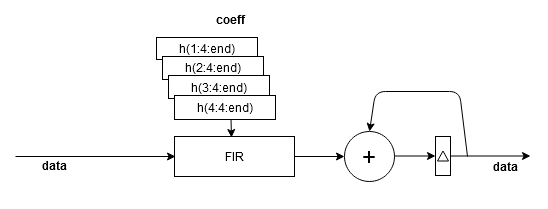• Architecture 5 — Fully parallel polyphase interleaved filter bank (vector)

The diagram shows the polyphase filter bank for an input vector size smaller than the decimation factor. This filter has an input vector of four values and DecimationFactor is set to 8. Each of the four subfilters has two sets of coefficients interleaved in time. The filter uses InputSize*FilterLength/DecimationFactor multipliers.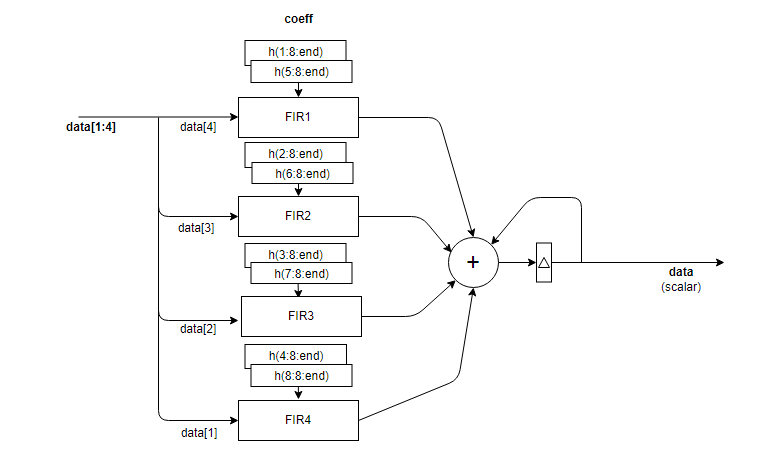• Architecture 6 — Fully parallel frame-based filter bank

With an input vector size greater than the decimation factor, the block implements decimation factor subfilters, each with frame-based input of VectorSize/DecimationFactor samples. The output vector has VectorSize/DecimationFactor samples. The filter uses InputSize*FilterLength/DecimationFactor multipliers.Each subfilter is implemented with a Discrete FIR Filter block. The adder at the output is pipelined to accommodate higher synthesis frequencies. For architecture details, see FIR Filter Architectures for FPGAs and ASICs.

Note

The output of the FIR Decimator block does not match the output of the FIR Decimation block from DSP System Toolbox™ sample-for-sample. This difference is mainly because of the phase in which the samples are applied across the subfilters. To match the FIR Decimation block, apply Decimation factor – 1 zeros to the FIR Decimator block at the start of the data stream.

The FIR Decimation block also uses slightly different data types for full-precision calculations. The different data types can also introduce differences in output values if the values overflow the internal data types.

## Version History

Introduced in R2020b

expand all